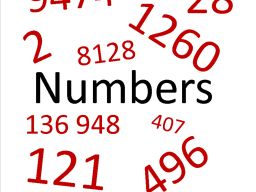# Fifteen 5

Fifteen more than five times a number equals the difference between -57 and three times the number. Find this integer number.

x =  -36

### Step-by-step explanation:Did you find an error or inaccuracy? Feel free to write us. Thank you!

Tips for related online calculators
Do you have a linear equation or system of equations and looking for its solution? Or do you have a quadratic equation?